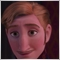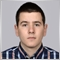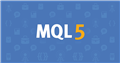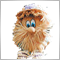# Help with CopyHigh19

I need the High for PastBars

BarPos is always correct giving me the position of the highest high during the last PastBars but the Highest Bar figure BarHigh2 is always wrong.

Can some one explain why CopyHigh is not working for me please?

int PastBars = 10;  // Number of past bars to find highest bar.

int OldBars = PastBars -1;

double BarHigh = 0;     // Highest bar value from PastBars range.

double BarHigh2 = 100;     // Highest bar value from PastBars range.

int BarPos = 100;

double High[];

ArraySetAsSeries(High,true);

int copied=CopyHigh(NULL,0,0,PastBars,High);

if(copied>0 && (OldBars)<copied) BarHigh2=High[OldBars];

BarPos = (ArrayMaximum(High,0,PastBars))+1;

Comment("Highest bar value from prev: ",PastBars," bars is bar: ",BarPos," and value: ",BarHigh2);7174

hrhsii:

I need the High for PastBars

BarPos is always correct giving me the position of the highest high during the last PastBars but the Highest Bar figure BarHigh2 is always wrong.

Can some one explain why CopyHigh is not working for me please?

int PastBars = 10;  // Number of past bars to find highest bar.

int OldBars = PastBars -1;

double BarHigh = 0;     // Highest bar value from PastBars range.

double BarHigh2 = 100;     // Highest bar value from PastBars range.

int BarPos = 100;

double High[];

ArraySetAsSeries(High,true);

int copied=CopyHigh(NULL,0,0,PastBars,High);

if(copied>0 && (OldBars)<copied) BarHigh2=High[OldBars];

BarPos = (ArrayMaximum(High,0,PastBars))+1;

Comment("Highest bar value from prev: ",PastBars," bars is bar: ",BarPos," and value: ",BarHigh2);

hey , i think your issue is this

`CopyHigh(NULL,0,0,PastBars,High);`

the High array so far is with size 0 , you need to resize it to PastBars size in order to work.

So, Resize the array before the CopyHigh and you will be alright19

Stanislav Ivanov:

hey , i think your issue is this

the High array so far is with size 0 , you need to resize it to PastBars size in order to work.

So, Resize the array before the CopyHigh and you will be alright

Thanks for the reply, I'm not sure how to resize the array, but I got it working, i needed the Highs and Lows to convert an MT4 Oscar Indicator to work in MT5 what i did was:

```double Y = 50;
for (int i=Bars(NULL,0)-(iLookBack); i>=0; i--)  {       //loop the no. of bars in chart - lookback
double X = Y;                                      // X = the previous oscillator figure (Oscar)

copied=CopyHigh(NULL,0,i,iLookBack,High);
BarPos = (ArrayMaximum(High,0,iLookBack))+1;
BarHigh = High[BarPos-1];

copiedLow=CopyLow(NULL,0,i,iLookBack,Low);
BarPosLow = (ArrayMinimum(Low,0,iLookBack))+1;
BarLow = Low[BarPosLow-1];

double C = iClose(NULL,0,i);                             // C = current bar's closing price
double rough  = (C-BarLow)/(BarHigh-BarLow)*100;
Y = ((X/3)*2) + (rough/3);
OscBuffer1[i] = MathRound(Y);
}```

To draw the simple Oscar indicator I used the Oscar formula which needs the Highest and Lowest Closing price in the last 8 bars (iLookBack).

I then looped round the number of bars on the chart and plot the result of the oscar formula Y.

With the start position of i, the array High seems to get filled and BarLow and BarHigh are now correct.

``` copiedLow=CopyLow(NULL,0,i,iLookBack,Low);
BarPosLow = (ArrayMinimum(Low,0,iLookBack))+1;
BarLow = Low[BarPosLow-1];   ```

I am new to C++ and MT5 so am not really sure how it is working.

My only problem now is the indicator is 0 for the amount of bars i have to look back making it useless.

With a little testing using the comment, i can see the last entry in OSCBuffer1 is 81, so i do not understand why 0 is being plotted.

```//+------------------------------------------------------------------+
//|                                                  Oscar_9_tst.mq5 |
//|                        Copyright 2020, MetaQuotes Software Corp. |
//|                                             https://www.mql5.com |
//+------------------------------------------------------------------+
#property version   "1.00"
#property indicator_separate_window
//#property indicator_chart_window
#property indicator_minimum -100
#property indicator_maximum 100
#property indicator_level1 75
#property indicator_level2 25
#property indicator_levelcolor DarkSlateGray

#property indicator_buffers 1
#property indicator_plots 1
//--- the OSCAR plot
#property indicator_type1 DRAW_LINE
#property indicator_label1  "OscarAvg"      // Name of a plot for the Data Window
#property indicator_color1 clrBlue      // Line color
#property indicator_style1 STYLE_SOLID // Line style
#property indicator_width1 1           // Line Width

//---- input parameters
input int iLookBack = 8;               //No. of bars to look back
input int OscarAve = 5;

string  IndiName1;

//---- buffers
double OscBuffer1[];

//+------------------------------------------------------------------+
//| Custom indicator initialization function                         |
//+------------------------------------------------------------------+
int OnInit()
{
//--- indicator buffers mapping
SetIndexBuffer(0,OscBuffer1,INDICATOR_DATA);
IndiName1 = "Oscar(" + iLookBack + ")";
IndicatorSetString(INDICATOR_SHORTNAME,IndiName1);

//--- sets first bar from what index will be drawn
PlotIndexSetInteger(0,PLOT_DRAW_BEGIN,OscarAve);
//---- line shifts when drawing
PlotIndexSetInteger(0,PLOT_SHIFT,0);

//---
return(INIT_SUCCEEDED);
}

//+------------------------------------------------------------------+
//| Custom indicator iteration function                              |
//+------------------------------------------------------------------+
int OnCalculate(const int rates_total,
const int prev_calculated,
const datetime &time[],
const double &open[],
const double &high[],
const double &low[],
const double &close[],
const long &tick_volume[],
const long &volume[],
{
//---
int OldBars = iLookBack -1;
double BarHigh = 0;      // Highest bar value from iLookBack range.
double BarLow = 100;     // Lowest bar value from PastBars range.
int BarPos = 100;
int BarPosLow = 100;
int copied = 0;
int copiedLow = 0;
double High[];
double Low[];
ArraySetAsSeries(High,true); // Highest price array
ArraySetAsSeries(Low,true);  // Lowest price array

double Y = 50;
int imax = Bars(NULL,0)-(iLookBack);
for (int i=Bars(NULL,0)-(iLookBack); i>=0; i--)  {       //loop the no. of bars in chart - lookback
double X = Y;                             // X = the previous oscillator figure (Oscar)

copied=CopyHigh(NULL,0,i,iLookBack,High);
BarPos = (ArrayMaximum(High,0,iLookBack))+1;
BarHigh = High[BarPos-1];

copiedLow=CopyLow(NULL,0,i,iLookBack,Low);
BarPosLow = (ArrayMinimum(Low,0,iLookBack))+1;
BarLow = Low[BarPosLow-1];

double C = iClose(NULL,0,i);                             // C = current bar's closing price
double rough  = (C-BarLow)/(BarHigh-BarLow)*100;
Y = ((X/3)*2) + (rough/3);
OscBuffer1[i] = MathRound(Y);
}

double TodayC = iClose(NULL,0,0);
Comment("first Y = ",Y,",Buffer, ",OscBuffer1 ,", Highestt bar from: ",iLookBack," bars is bar: ",BarPos," and value: ",BarHigh, "Lowest bar from: ",iLookBack," bars is bar: ",BarPosLow," and value: ",BarLow);
//Comment ("Today's close: ", TodayC, "MA: ",maVal,", ",maVal);

//--- return value of prev_calculated for next call
return(rates_total);
}```Documentation on MQL5: Constants, Enumerations and Structures / Chart Constants / Chart Properties
• www.mql5.com
Price chart drawing. If false, drawing any price chart attributes is disabled and all chart border indents are eliminated, including time and price scales, quick navigation bar, Calendar event labels, trade labels, indicator and bar tooltips, indicator subwindows, volume histograms, etc. Scrolling the chart horizontally using the left mouse...
Files:Moderator
206919

hrhsii :

Thanks for the reply, I'm not sure how to resize the array, but I got it working, i needed the Highs and Lows to convert an MT4 Oscar Indicator to work in MT5 what i did was:

To draw the simple Oscar indicator I used the Oscar formula which needs the Highest and Lowest Closing price in the last 8 bars (iLookBack).

I then looped round the number of bars on the chart and plot the result of the oscar formula Y.

With the start position of i, the array High seems to get filled and BarLow and BarHigh are now correct.

I am new to C++ and MT5 so am not really sure how it is working.

My only problem now is the indicator is 0 for the amount of bars i have to look back making it useless.

With a little testing using the comment, i can see the last entry in OSCBuffer1 is 81, so i do not understand why 0 is being plotted.

You have

``` int OnCalculate ( const int rates_total,
const int prev_calculated,
const datetime &time[],
const double &open[],
const double &high[],
const double &low[],
const double &close[],
const long &tick_volume[],
const long &volume[],

why do you use THIS:

```   int imax = Bars ( NULL , 0 )-(iLookBack);
for ( int i= Bars ( NULL , 0 )-(iLookBack); i>= 0 ; i--)  {       //loop the no. of bars in chart - lookback
double X = Y;                             // X = the previous oscillator figure (Oscar)

copied= CopyHigh ( NULL , 0 ,i,iLookBack,High);
BarPos = ( ArrayMaximum (High, 0 ,iLookBack))+ 1 ;
BarHigh = High[BarPos- 1 ];

copiedLow= CopyLow ( NULL , 0 ,i,iLookBack,Low);
BarPosLow = ( ArrayMinimum (Low, 0 ,iLookBack))+ 1 ;
BarLow = Low[BarPosLow- 1 ];

double C = iClose ( NULL , 0 ,i);                             // C = current bar's closing price
double rough  = (C-BarLow)/(BarHigh-BarLow)* 100 ;
Y = ((X/ 3 )* 2 ) + (rough/ 3 );
```

- such work with indicators is a mistake.

It is still not clear what exactly you need. Try to ask your question in the form of a picture.19

You have

why do you use THIS:

- such work with indicators is a mistake.

It is still not clear what exactly you need. Try to ask your question in the form of a picture.

Thank you Vladimir, you have been very helpful. The Oscar indicator from MT4 is now working in MT5.

```//+------------------------------------------------------------------+
//|                                                  Oscar_9_tst.mq5 |
//|                        Copyright 2020, MetaQuotes Software Corp. |
//|                                             https://www.mql5.com |
//+------------------------------------------------------------------+
#property version   "1.00"
#property indicator_separate_window
//#property indicator_chart_window
#property indicator_minimum 0
#property indicator_maximum 100
#property indicator_level1 75
#property indicator_level2 25
#property indicator_levelcolor DarkSlateGray

#property indicator_buffers 1
#property indicator_plots 1
//--- the OSCAR plot
#property indicator_type1 DRAW_LINE
#property indicator_label1  "OscarAvg"      // Name of a plot for the Data Window
#property indicator_color1 clrBlue      // Line color
#property indicator_style1 STYLE_SOLID // Line style
#property indicator_width1 1           // Line Width

//---- input parameters
input int iLookBack = 8;               //No. of bars to look back
input int OscarAve = 5;

string  IndiName1;

//---- buffers
double OscBuffer1[];

//+------------------------------------------------------------------+
//| Custom indicator initialization function                         |
//+------------------------------------------------------------------+
int OnInit()
{
//--- indicator buffers mapping
SetIndexBuffer(0,OscBuffer1,INDICATOR_DATA);
IndiName1 = "Oscar(" + iLookBack + ")";
IndicatorSetString(INDICATOR_SHORTNAME,IndiName1);

//--- sets first bar from what index will be drawn
//   PlotIndexSetInteger(0,PLOT_DRAW_BEGIN,OscarAve);
//---- line shifts when drawing
//   PlotIndexSetInteger(0,PLOT_SHIFT,0);

//---
return(INIT_SUCCEEDED);
}

//+------------------------------------------------------------------+
//| Custom indicator iteration function                              |
//+------------------------------------------------------------------+
int OnCalculate(const int rates_total,
const int prev_calculated,
const datetime &time[],
const double &open[],
const double &high[],
const double &low[],
const double &close[],
const long &tick_volume[],
const long &volume[],
{
//---
double BarHigh = 0;                                           // Highest bar value from iLookBack range.
double BarLow = 0;                                            // Lowest bar value from iLookBack range.
int chartBars = rates_total-1;
double OscarVal = 50;

//--- set direct indexing
ArraySetAsSeries(high,true);
ArraySetAsSeries(low,true);
ArraySetAsSeries(open,true);
ArraySetAsSeries(close,true);

for (int i = chartBars; i>=0; i--)  {                           //loop the no. of bars in chart - lookback
double X = OscarVal;                                         // X = the previous oscillator figure (Oscar)
if (i < (rates_total - iLookBack)){
BarHigh = high[(ArrayMaximum(high,i,iLookBack))];         // Highest bar value from iLookBack range.
BarLow = low[(ArrayMinimum(low,i,iLookBack))];            // Lowest bar value from iLookBack range.
double C = close[i];                                      // C = current bar's closing price
double rough  = (C-BarLow)/(BarHigh-BarLow)*100;
OscarVal = ((X/3)*2) + (rough/3);
}
OscBuffer1[chartBars-i] = OscarVal;

}

Comment(" First Oscar value = ",OscarVal,", OscBuffer1= ",OscBuffer1 ,", Highest bar within: ",iLookBack," bars is: ",BarHigh, "Lowest bar value within: ",iLookBack," bars is: ",BarLow);

//--- return value of prev_calculated for next call
return(rates_total);
}```

I was loading the Buffer for drawing using an array in reverse, that is why the output was wrong, i have now corrected it and the Oscar line is now correct. Please see the image attached.

How do i add a Moving average line?

I want to use the crossover as a signal later, but for now i just want to plot the moving average (5) with the Oscar line.

Files:
Oscar-8bar2.JPG 219 kb